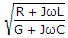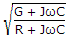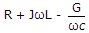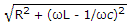# Electronics and Communication Engineering - Electromagnetic Field Theory

11.
In order to receive a vertical polarized wave, the conductor of the dipole should be mounted
horizontally
vertically
at an angle of 45°
at an angle of 90°
Explanation:
No answer description is available. Let's discuss.

12.
VSWR can have any value between
0 and 1
- 1 to + 1
0 to ∞
1 to ∞
Explanation:
No answer description is available. Let's discuss.

13.
The input impedance for folded dipole antenna (two half dipole) is
73 n2
377 n2
73 n
377 n
Explanation:
No answer description is available. Let's discuss.

14.
What is the characteristic impedance Z0 of a line having R, inductance L, capacitance C and conductance G?